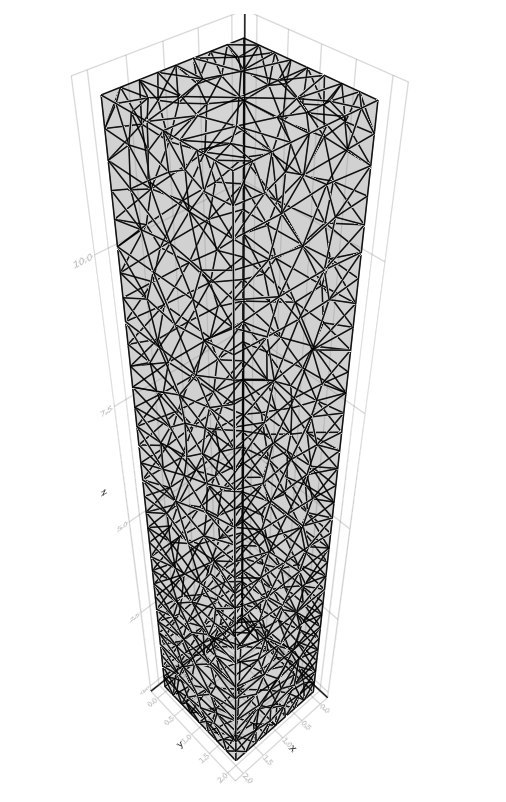16

3

5

3

# TetGen

The `TetGen.jl` package is a Julia wrapper for the C++ project TetGen. This wrapper enables TetGen based tetrahedral meshing, and (constrained) 3D Delaunay and Voronoi tesselation.

## Example

``````
points = zeros(8 * 3)
points[[4, 7, 8, 11]]  .= 2;  # node 2.
# Set node 5, 6, 7, 8.
for i in 4:7
points[i * 3 + 1] = points[(i - 4) * 3 + 1];
points[i * 3 + 1 + 1] = points[(i - 4) * 3 + 1 + 1];
points[i * 3 + 2 + 1] = 12;
end

# Facet 1. The leftmost JLFacet.
polygons = [
JLPolygon(Cint[1:4;]),
JLPolygon(Cint[5:8;]),
JLPolygon(Cint[1,5,6,2]),
JLPolygon(Cint[2,6,7,3]),
JLPolygon(Cint[3, 7, 8, 4]),
JLPolygon(Cint[4, 8, 5, 1])
]

facetlist = JLFacet.(polygons)

facetmarkerlist = Cint[-1, -2, 0, 0, 0, 0]

tio = TetgenIO(
collect(reinterpret(Point{3, Float64}, points)),
facets = facetlist,
facetmarkers = facetmarkerlist,
)

result = tetrahedralize(tio, "vpq1.414a0.1")
``````

Plotted with Makie:02/09/2015

9 days ago

44 commits